# NCERT Solutions - Continuity & Differentiability, Exercise 5.2 JEE Notes | EduRev

## JEE : NCERT Solutions - Continuity & Differentiability, Exercise 5.2 JEE Notes | EduRev

The document NCERT Solutions - Continuity & Differentiability, Exercise 5.2 JEE Notes | EduRev is a part of the JEE Course Mathematics (Maths) Class 12.
All you need of JEE at this link: JEE

Continuity & Differentiability

Question 1: Differentiate the functions with respect to x.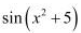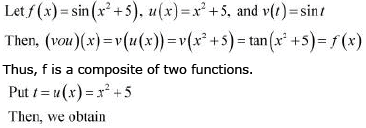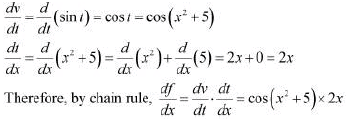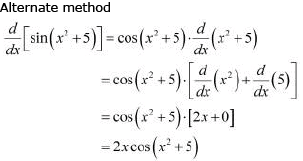Question 2: Differentiate the functions with respect to x. cos(sinx)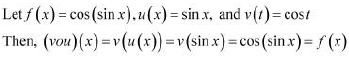Thus, f is a composite function of two functions.

Put t = u (x) = sin x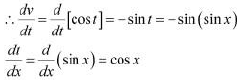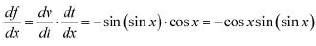By chain rule,
Alternate method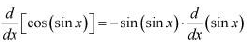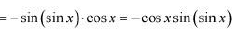Question 3: Differentiate the functions with respect to x.

sin(ax + b)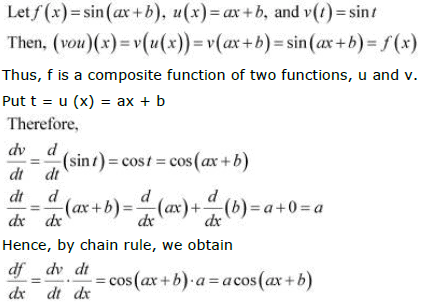Alternate method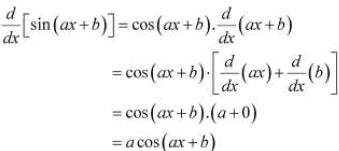Question 4: Differentiate the functions with respect to x.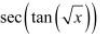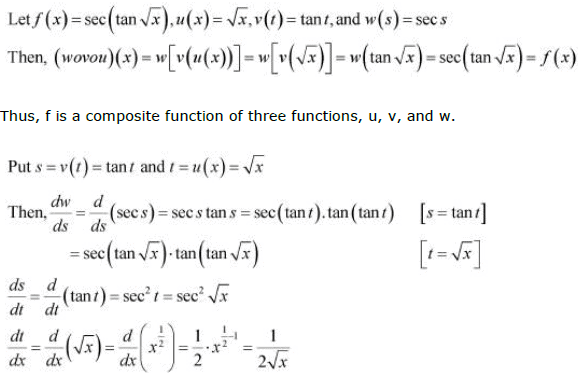Hence, by chain rule, we obtain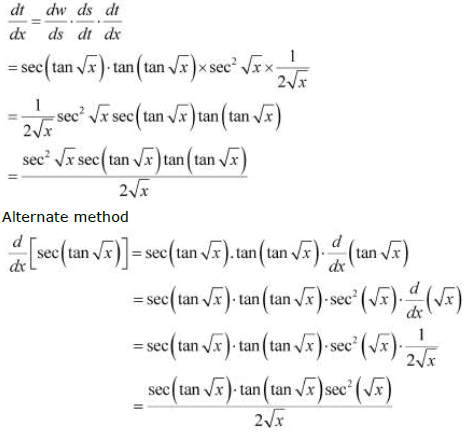Question 5: Differentiate the functions with respect to x.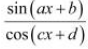The given function is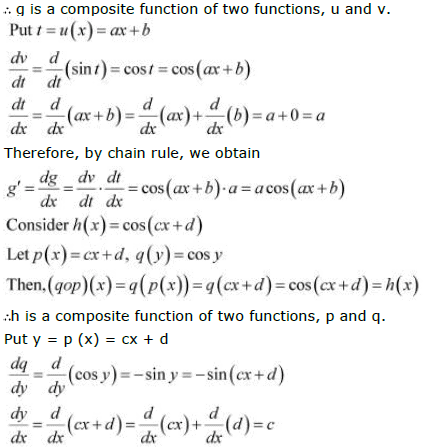Put y = p (x) = cx + d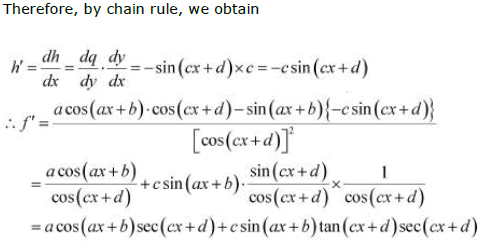Question 6: Differentiate the functions with respect to x.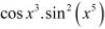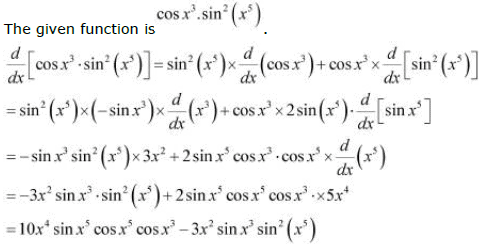Question 7:  Differentiate the functions with respect to x.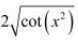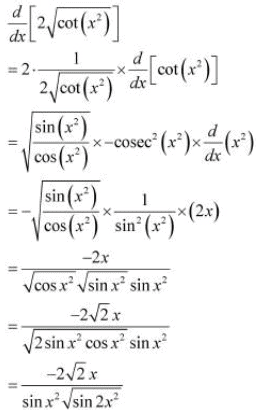Question 8: Differentiate the functions with respect to x.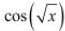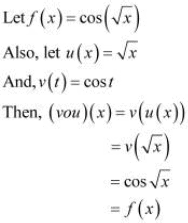Clearly, f is a composite function of two functions, u and v, such that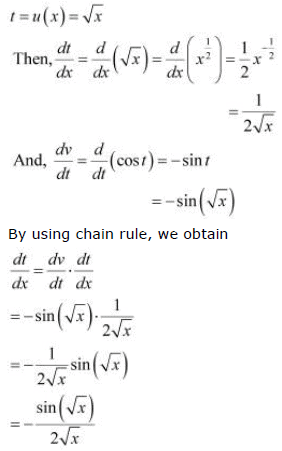Alternate method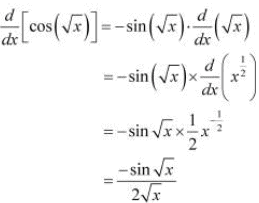Question 9:
Prove that the function f given by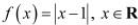is not differentiable at x = 1.

The given function isIt is known that a function f is differentiable at a point x = c in its domain if both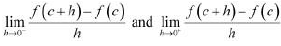are finite and equal.

To check the differentiability of the given function at x = 1,

consider the left hand limit of f at x = 1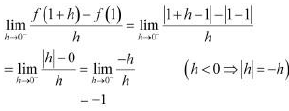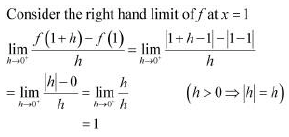Since the left and right hand limits of f at x = 1 are not equal, f is not differentiable at x = 1

Question 10:
Prove that the greatest integer function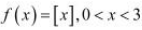defined by is not differentiable at x = 1 and x = 2.

The given function f isIt is known that a function f is differentiable at a point x = c in its domain if both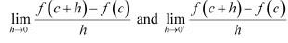are finite and equal.

To check the differentiability of the given function at x = 1, consider the left hand limit  of f at x = 1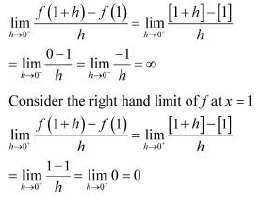Since the left and right hand limits of f at x = 1 are not equal, f is not differentiable at x = 1

To check the differentiability of the given function at x = 2, consider the left hand limit of f at x = 2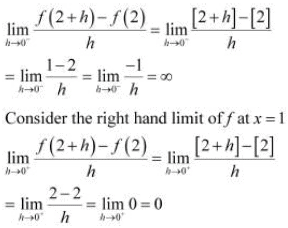Since the left and right hand limits of f at x = 2 are not equal, f is not differentiable at x = 2

Offer running on EduRev: Apply code STAYHOME200 to get INR 200 off on our premium plan EduRev Infinity!

## Mathematics (Maths) Class 12

209 videos|222 docs|124 tests

,

,

,

,

,

,

,

,

,

,

,

,

,

,

,

,

,

,

,

,

,

,

,

,

;